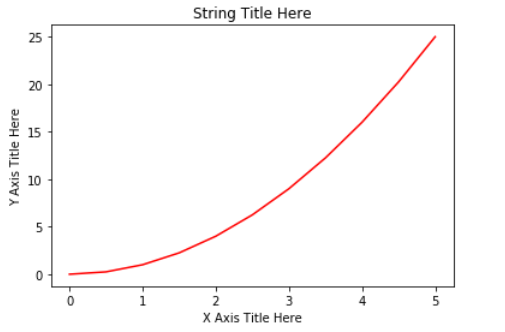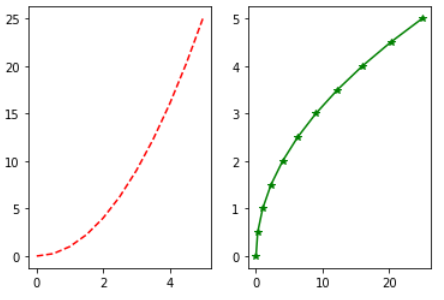1618312517

# Higher Physics Data Analysis & Visualization Using Python(Matplotlib | Numpy) 🌌

Abstract:
Programming is influencing all disciplines and helping scientiests discover in the field of Science and Mathematics with the help of technology. The concept of Simple Harmonic Motion is uded to create graph of waves using Python(Numpy | Matplotlib | Scipy) where the user can make changes to the amplitutde, initial phase and frequency of the wave. User can also add a decay or damping constant. User can also plot any SHM graphs using this program. As a demonstration spring simulation has been given in .py format.

www.github.com/qxresearch/Simple-Harmonic-Motion

The projects Content:

1. Program SHM-1.py is console based user defined program where user can change: frequency, apmlitude and intial phase
2. Program SHM-2.py will plot a spring simulation
3. Download the code from GitHub(www.github.com/qxresearch/Simple-Harmonic-Motion) and run(double click) the .py file to plot the graph.

📑 If you think this project has helped you learn something new you can give a star on GitHub
📑 If you think it’s a bad project do point out ‘why’ and spam the issue section 🚩

#qxresearch #physics #python #numpy #matplotlib #data-analysis

## Buddha Community1618315215

if you want different numpy matplotlib tutorial, let me know in the comment section. Keep learning :)

1618312517

## Higher Physics Data Analysis & Visualization Using Python(Matplotlib | Numpy) 🌌

Abstract:
Programming is influencing all disciplines and helping scientiests discover in the field of Science and Mathematics with the help of technology. The concept of Simple Harmonic Motion is uded to create graph of waves using Python(Numpy | Matplotlib | Scipy) where the user can make changes to the amplitutde, initial phase and frequency of the wave. User can also add a decay or damping constant. User can also plot any SHM graphs using this program. As a demonstration spring simulation has been given in .py format.

www.github.com/qxresearch/Simple-Harmonic-Motion

The projects Content:

1. Program SHM-1.py is console based user defined program where user can change: frequency, apmlitude and intial phase
2. Program SHM-2.py will plot a spring simulation
3. Download the code from GitHub(www.github.com/qxresearch/Simple-Harmonic-Motion) and run(double click) the .py file to plot the graph.

📑 If you think this project has helped you learn something new you can give a star on GitHub
📑 If you think it’s a bad project do point out ‘why’ and spam the issue section 🚩

#qxresearch #physics #python #numpy #matplotlib #data-analysis1619518440

## top 30 Python Tips and Tricks for Beginners

Welcome to my Blog , In this article, you are going to learn the top 10 python tips and tricks.

### 8) Check The Memory Usage Of An Object.

#python #python hacks tricks #python learning tips #python programming tricks #python tips #python tips and tricks #python tips and tricks advanced #python tips and tricks for beginners #python tips tricks and techniques #python tutorial #tips and tricks in python #tips to learn python #top 30 python tips and tricks for beginners1594088160

## Data Visualization With Python: Matplotlib

Data visualization is the graphical representation of data in a graph, chart or other visual formats. It shows relationships of the data with images.

Python offers multiple graphics libraries, with which you can create interactive, live or highly customizable plots with the given data.

To get a little overview here are a few popular plotting libraries:

In this article, we will learn about creating a different type of plots using the Matplotlib library.

Matplotlib is the most popular plotting library for python, which was designed to have a similar feel to MATLAB’s graphical plotting. It gives you control over every aspect of a plot.

Matplotlib allows you to create reproducible figures using a few lines of code. Let’s learn how to use it! I also encourage you to explore: http://matplotlib.org/.

## Installing Matplotlib

Install it with pip or conda at your command line or the terminal with:-

``````pip install matplotlib
OR
conda install matplotlib
``````

To quickly get started with Matplotlib without installing anything on your local machine, check out Google Colab. It provides Jupyter Notebooks hosted on the cloud for free which are associated with your Google Drive account and it comes with all the important packages pre-installed.

## Necessary Imports

`pyplot` is a module of Matplotlib that makes this library work like MATLAB. Import the `matplotlib.pyplot` module under the name `plt` (the tidy way):

``````import matplotlib.pyplot as plt
import numpy as np # for working with arrays
``````

## Making a Simple Plot

We pass two NumPy arrays(x and y) and ‘r’ as arguments to Pyplot’s `plot()` function. Here ‘r’ is for red colour, x elements will appear on x-axis and y elements will appear on the y-axis.

``````import matplotlib.pyplot as plt
import numpy as np

x = np.array([0, 0.5, 1, 1.5, 2, 2.5, 3, 3.5, 4, 4.5, 5])
y = x ** 2 # y is now a list with elements of x to the power 2

plt.plot(x, y, 'r')
plt.xlabel('X Axis Title Here')
plt.ylabel('Y Axis Title Here')
plt.title('String Title Here')
plt.show()
# The plot below is the output of this program.
``````Creating Multiple Plots on The Same Canvas

`subplot()`: a method of pyplot, divides the canvas into `nrows` x `ncols` parts and using `plot_number` argument you can choose the plot.

Syntax: `subplot(nrows, ncols, plot_number)`

In the below example, using `plt.plot(x, y, 'r--’)`we plot a red coloured graph with line style ‘- -’ between x and y at plot_number=1.

``````import matplotlib.pyplot as plt
import numpy as np

x = np.array([0, 0.5, 1, 1.5, 2, 2.5, 3, 3.5, 4, 4.5, 5])
y = x ** 2
plt.subplot(1,2,1) # subplot(nrows, ncols, plot_number)
plt.plot(x, y, 'r--') # r-- meaning colour red with -- pattern
plt.subplot(1,2,2)
plt.plot(y, x, 'g*-') # g*- meaning colour green with *- pattern
# The plot below is the output of this program.
``````For making it more simple `subplots()` method can be used instead of `subplot()`. You will see its example in “Creating Multiple plots on The Same Canvas” under “Matplotlib Object-Oriented Method”.

#data-science #matplotlib #data-visualization #python #plotting-data #data analysis1617988080

## How To Blend Data in Google Data Studio For Better Data Analysis

Using data to inform decisions is essential to product management, or anything really. And thankfully, we aren’t short of it. Any online application generates an abundance of data and it’s up to us to collect it and then make sense of it.

Google Data Studio helps us understand the meaning behind data, enabling us to build beautiful visualizations and dashboards that transform data into stories. If it wasn’t already, data literacy is as much a fundamental skill as learning to read or write. Or it certainly will be.

Nothing is more powerful than data democracy, where anyone in your organization can regularly make decisions informed with data. As part of enabling this, we need to be able to visualize data in a way that brings it to life and makes it more accessible. I’ve recently been learning how to do this and wanted to share some of the cool ways you can do this in Google Data Studio.

#google-data-studio #blending-data #dashboard #data-visualization #creating-visualizations #how-to-visualize-data #data-analysis #data-visualisation1620466520